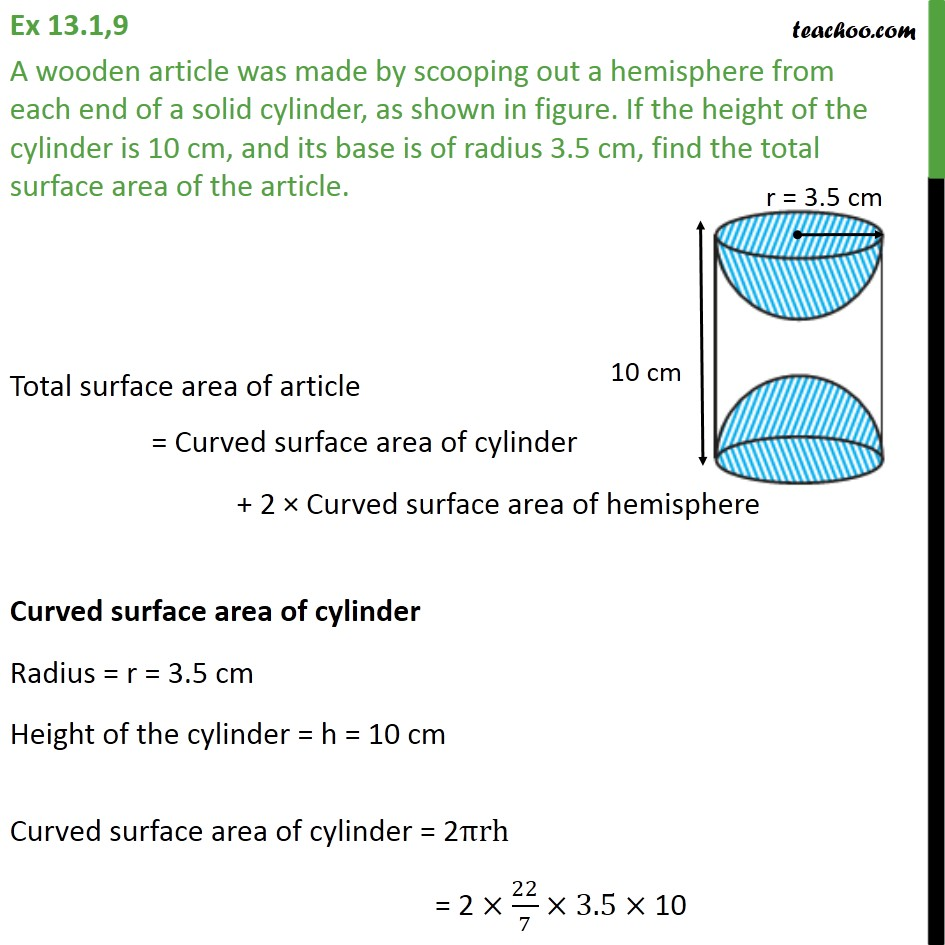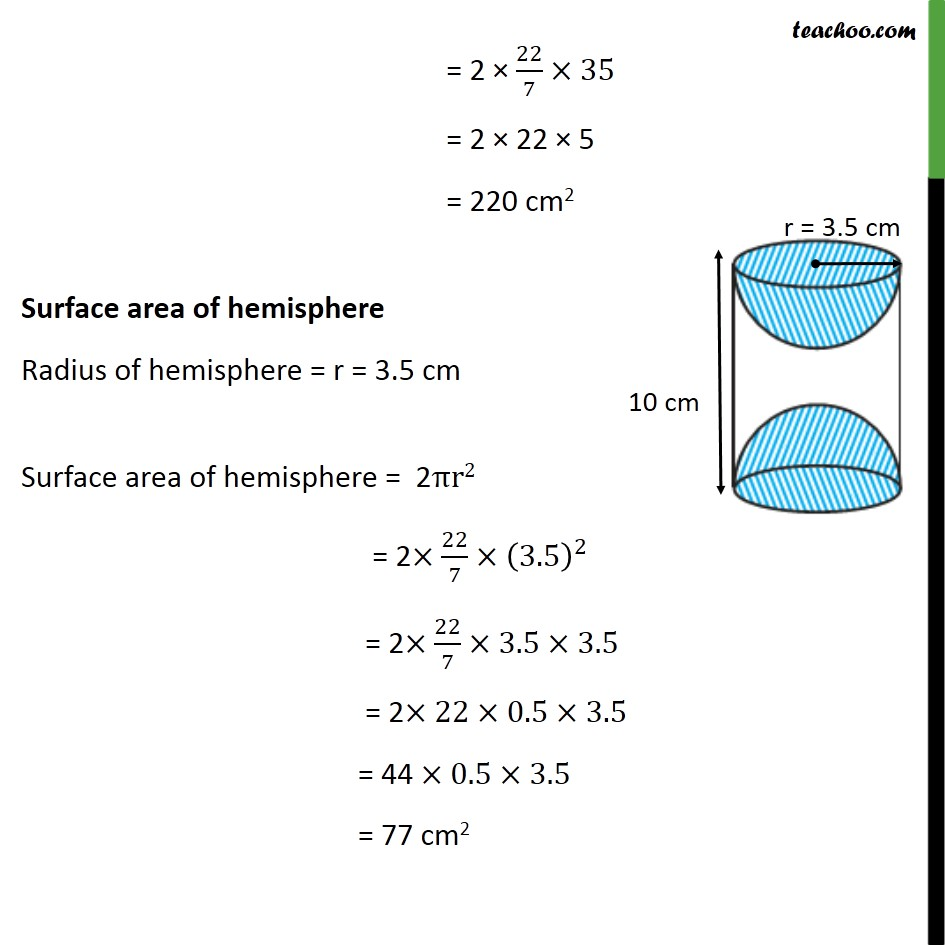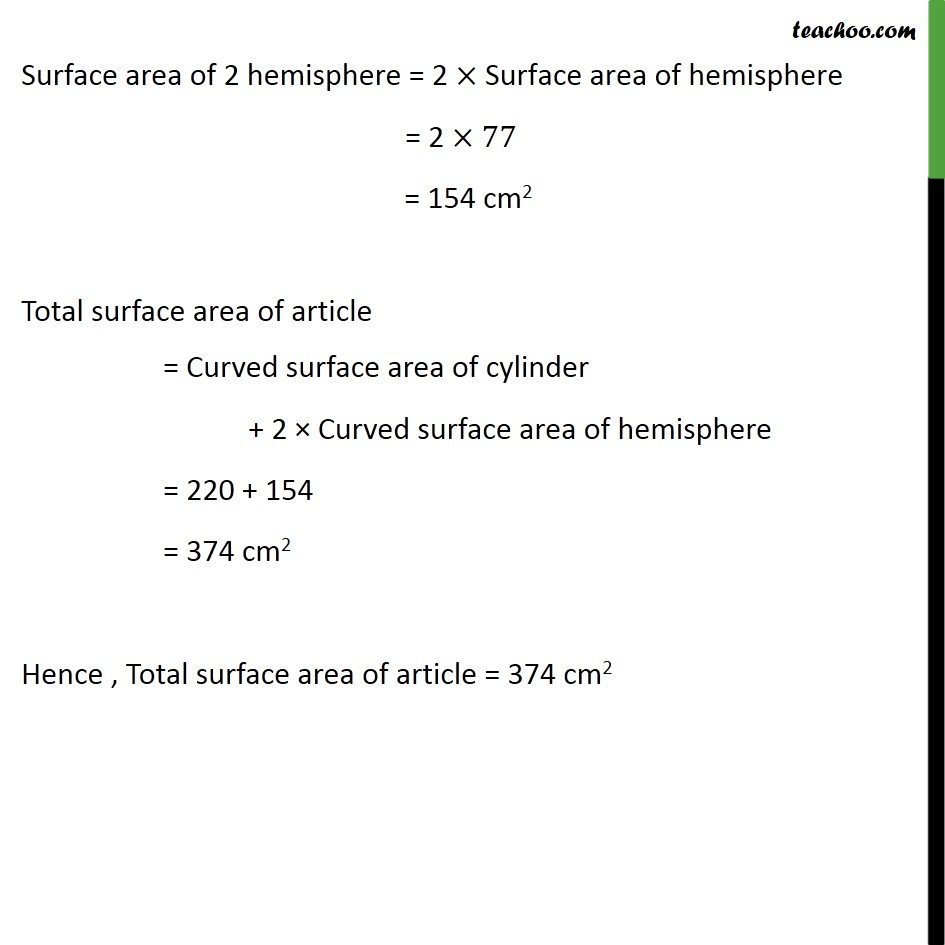1. Class 10
2. Important Questions for Exam - Class 10
3. Chapter 13 Class 10 Surface Areas and Volumes

Transcript

Ex 13.1,9 A wooden article was made by scooping out a hemisphere from each end of a solid cylinder, as shown in figure. If the height of the cylinder is 10 cm, and its base is of radius 3.5 cm, find the total surface area of the article. Total surface area of article = Curved surface area of cylinder + 2 × Curved surface area of hemisphere Curved surface area of cylinder Radius = r = 3.5 cm Height of the cylinder = h = 10 cm Curved surface area of cylinder = 2πrh = 2 ×22/7×3.5× 10 = 2 × 22/7×35 = 2 × 22 × 5 = 220 cm2 Surface area of hemisphere Radius of hemisphere = r = 3.5 cm Surface area of hemisphere = 2πr2 = 2×22/7×(3.5)^2 = 2×22/7×3.5×3.5 = 2×22×0.5×3.5 = 44 ×0.5×3.5 = 77 cm2 Surface area of 2 hemisphere = 2 ×"Surface area of hemisphere" = 2 ×77 = 154 cm2 Total surface area of article = Curved surface area of cylinder + 2 × Curved surface area of hemisphere = 220 + 154 = 374 cm2 Hence , Total surface area of article = 374 cm2

Chapter 13 Class 10 Surface Areas and Volumes

Class 10
Important Questions for Exam - Class 10